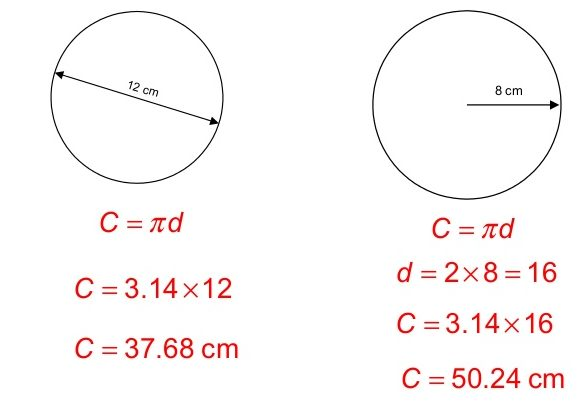How to find circumference

Want create site? Find Free WordPress Themes and plugins.

How to find circumference? A circle is an important shape in the mathematical world. There are a lot of problems involving it and it has many parts. It has a radius, diameter, circumference, center, arc, sector, segment etc. To find out each of them, there are different formula and techniques.  You will have to know the most common ones, that is the area of the circle.

Related articles about How to find circumference

How to find the area of a circle

How to find the area of a triangle

How to find the area of a trapezoid

How to find the circumference of a circleHow to find circumference

Let us see how we find the circumference. It is the outer part of the circle that gives it the round shape. You will have to know the diameter in order to find the circumference. The diameter is the line from one part of the circle to the other part and crossing through the center. The formula of circumference is circumference=2πr or πd. Based on the question, you decide which one to utilize.

Example: Find the circumference of a circle whose diameter is 5 inches.

Circumference = π x d (the question says diameter so we picked this one)

= 3.14 X 5 inches

= 15.7 inches (The unit will be the same as the unit of the diameter, for cm the result will be in cm)

The units are normally centimeters, meters or inches. If you order a pizza of 12 inches, that is the value of the diameter. The value of pi is 3.14159 but here we are using 3.14

To remember the formula or if you want to know how it originates, then read on:

π = Circumference / Diameter

Therefore, it is 2r.

Now, multiply 2r with π and you will get the formula of the circumference.

People confuse the area of the circle with the circumference.

Look at the difference, one of them is about r² (area) while the other one is just the diameter which is 2r.

To remember them, you can start by memorizing them. Then, understand that the area is the square where is the circumference requires just the radius two time. You can rewrite the formula of circumference as

Circumference = 2 π r or π (r + r) AND Area = π r²

You can already see the difference and when you do the math, you will see that they are completely different.

Calculate the area and circumference of a circle with the radius 3 inches.

Area = π r²

= 3.14 x 3²

= 28.26 inches ² (The unit is inches’ square)

Circumference = 2 π r

C = 2 π r

= 2 x 3.14 x 3

= 18.84 inches

Now, you can tell that they are entirely different and avoid the confusion.

Tips

• If you are allowed to use calculators to solve this, consider purchasing one that has the built-in π so that you can solve the equations easily.

• Not every math problem will let you use pi according to your wish, you may have to use the fraction value which is

Did you find apk for android? You can find new Free Android Games and apps.

We will be happy to hear your thoughts

This site uses Akismet to reduce spam. Learn how your comment data is processed.